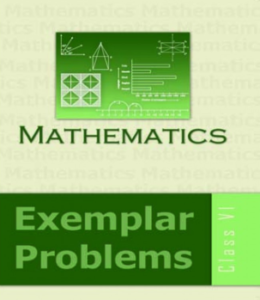# NCERT EXEMPLAR BOOK CLASS 6 Maths

## CBSE NCERT EXEMPLAR BOOK CLASS 6 Maths

NCERT Exemplar Books are important for students of Class 6. The NCERT books as a self-assessment guide to the students. There are questions which cover elementary concepts. Thereby, preparing students with in-depth knowledge. The NCERT Exemplar Book Class 6 Maths provides questions which are very practical oriented and to the point. The questions cover all concepts really well. NCERT Books are in accordance with the CBSE syllabus. Not only that, it is suitable for students of other Boards as well. hence, students learning basic concepts must try NCERT Exemplar Book Class 6 Maths.

Download free PDF files of NCERT Exemplar problems of Class 6 Maths.

## Why NCERT Exemplar Book Class 6 Maths?

NCERT Exemplar Class 6 Maths is considered an important study material for students of class 6. It is a known fact that students start to learn many new concepts from class 6 onwards. Which in turn, helps them to prepare well for the upcoming Board exams. NCERT Exemplar Book Class 6 Maths helps the students to develop those concepts starting from scratch. It introduces students to Number Systems, Algebra, Decimals, Fractions, Data handling, Mensuration, Ratio and Proportions and Geometry.

There are a total of 9 chapters in NCERT Exemplar Book Class 6 Maths. The students learn new concepts of Maths, which is interesting and fun too. With regular practice, students are sure to excel. The book comprises of Summary, Solved Exercises and Unsolved Exercises. The summary acts as a note for all important points of the chapters. It gives a brief description of the concepts. The Solved Exercises gives an idea to the students about the types of questions. The Unsolved Exercises lets them analyse their logical and problem-solving skills. Thus, NCERT Exemplar Book Class 6 Maths is a must for students of class 6.